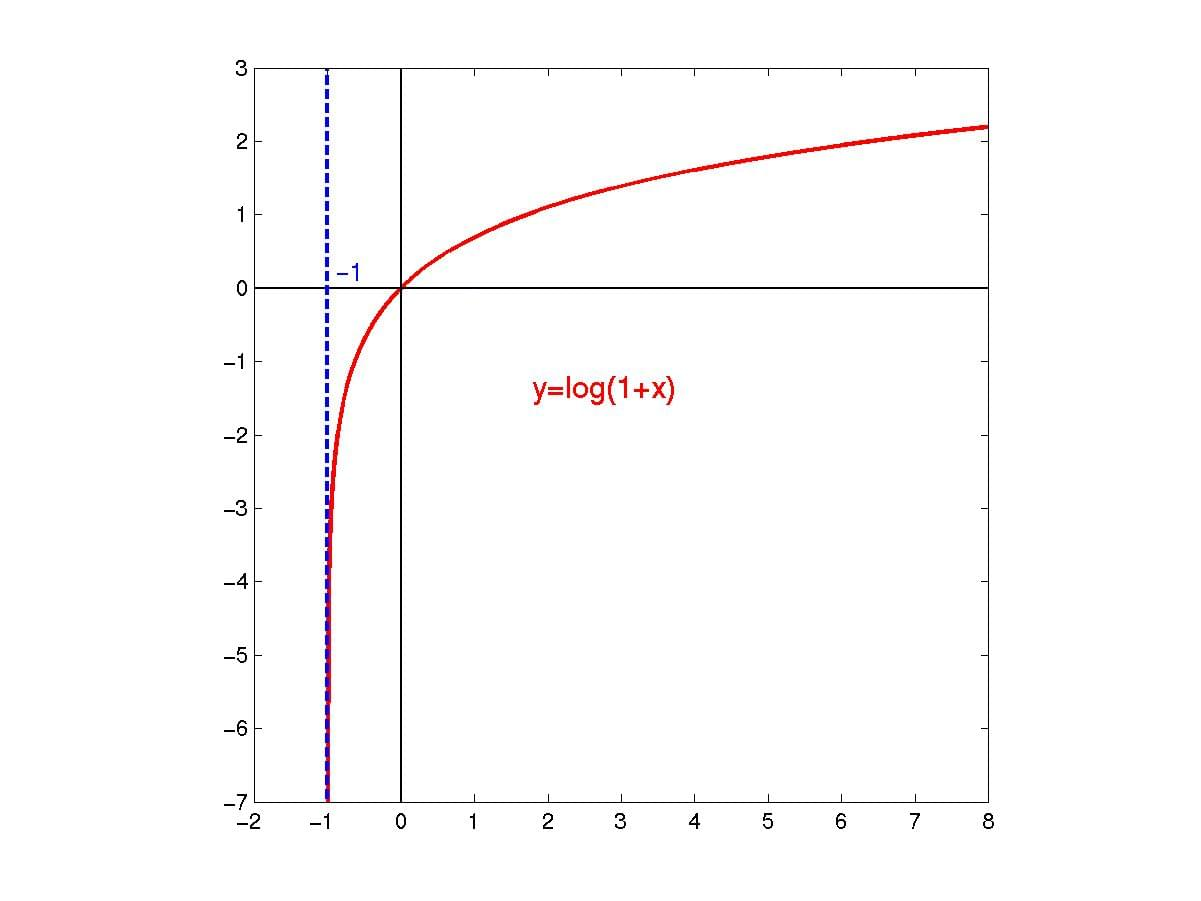# Math.log1p()

## 概述

`Math.log1p()` 函数返回一个数字加 1 后的自然对数 (底为 `E`), 既`log(x+1)`.

## 语法

```Math.log1p(x)
```

`x`

## 描述``````Math.log1p(Math.E-1)  // 1
Math.log1p(0)         // 0
Math.log1p("0")       // 0
Math.log1p(-1)        // -Infinity
Math.log1p(-2)        // NaN
Math.log1p("foo")     // NaN
``````

## 规范

Specification
ECMAScript Language Specification
# sec-math.log1p

## 浏览器兼容性

BCD tables only load in the browser